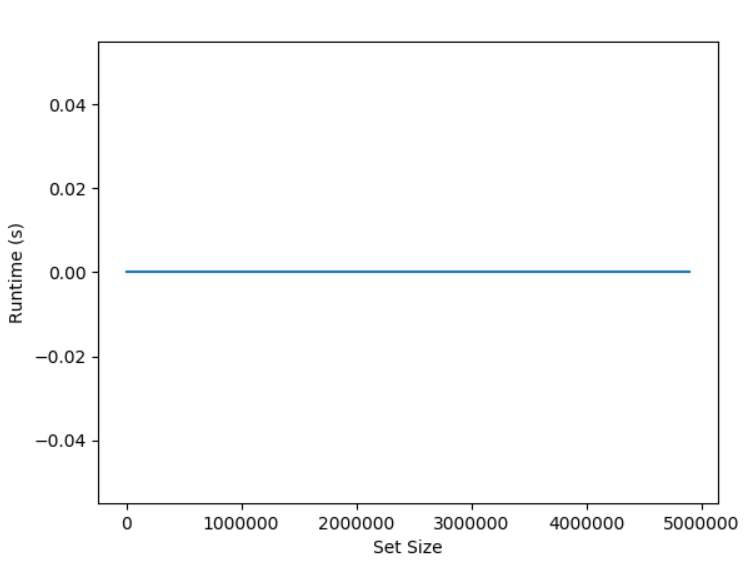# Python Set pop()

4/5 - (1 vote)

Python’s `set.pop()` method removes and returns an arbitrary element from the set. If no element is present — that is, you call it on an empty set — `set.pop()` raises a `KeyError`.

Here’s a minimal example where you remove a string element that happens to be `'Alice'` from the set by means of the `s.pop()` method:

```>>> s = {'Alice', 'Bob', 'Carl'}
>>> s.pop()
'Alice'```

## Syntax

Let’s dive into the formal syntax of the `set.pop()` method.

`set.pop()`

## Python Set pop() a Specific Element

? The `set.pop()` method doesn’t take an argument. If you still pass an argument, it raises a `TypeError: pop() takes no arguments (1 given)`. To fix this, don’t pass an argument into the method. If you need to remove a specific element from the set, use the `set.remove(x)` method, or, to avoid an error when the element to be removed is not present, use the `set.discard(x)` method.

```>>> s = {'Alice', 'Bob', 'Carl'}
>>> s.pop('Bob')
Traceback (most recent call last):
File "<pyshell#7>", line 1, in <module>
s.pop('Bob')
TypeError: pop() takes no arguments (1 given)

>>> s.remove('Bob')
>>> s
{'Alice', 'Carl'}```

## Return Value of Set pop()

The return value of `set.pop()` is an `object` that was stored as a set element. If the set is empty, it raises a `KeyError`.

## How to Fix KeyError: ‘pop from an empty set’

? The `set.pop()` method assumes that the set is non-empty. If you call the method on an empty set, it raises a `KeyError: 'pop from an empty set'`. To fix this, make sure that the set is non-empty before calling the pop() method or use the `set.discard()` method instead that doesn’t raise an error on a non-existent key.

```>>> set().pop()
Traceback (most recent call last):
File "<pyshell#11>", line 1, in <module>
set().pop()
KeyError: 'pop from an empty set'```

Here’s a code function `pop_no_error()` that takes a set argument and pops an arbitrary element if one exists. Otherwise it returns `None`:

```>>> def pop_no_error(my_set):
return my_set.pop() if my_set else None

>>> pop_no_error({1, 2, 3})
1
>>> pop_no_error(set())
>>> ```

## set.pop() vs set.remove()

The `set.pop()` method is similar to the `set.remove()` method. Both methods remove an element from the set. However, they’re quite different nonetheless.

? The difference between `set.pop()` and `set.remove()` is that the former removes and returns an arbitrary element whereas the latter removes a specific element and doesn’t return it.

You can see this in the following example:

```>>> s = {'Alice', 'Bob', 'Carl'}
>>> s.remove('Carl')
>>> s
{'Alice', 'Bob'}
>>> s.pop()
'Alice'
>>> s
{'Bob'}```

Please note that the remove method has no return value but side effects—the element `'Carl'` doesn’t appear in the set anymore. The pop method has a return value and has side effects as well—the element `'Alice'` doesn’t appear in the set anymore.

## Python Set Pop Multiple Elements

Problem Formulation: Given a set and an integer n. You want to remove and return (“pop”) n random elements from the set. How do you accomplish this?

? To pop multiple elements from a set, use the list comprehension expression `[s.pop() for i in range(n)]`. This assumes that the set has at least `n` elements, otherwise a `KeyError` will be raised.

Here’s the code:

```s = {'Alice', 'Bob', 'Carl', 'Liz', 'Ann'}
n = 3

def pop_n(s, n):
'''Remove and return n elements from s'''
if n>len(s):
return list(s)
return [s.pop() for i in range(n)]

print(pop_n(s, n))
# ['Ann', 'Liz', 'Bob']

print(s)
# {'Ann', 'Carl'}```

## What is the Time Complexity of set.pop()?

The runtime complexity of the `set.pop()` function on a set with n elements is O(1). So, Python’s `set.pop()` method has constant runtime complexity. It simply removes and returns the first element it encounters.

You can see this in the following simple experiment where we run the set method multiple times for an increasing number of set elements.Figure: The runtime (y-axis) is independent of the number of set elements (x-axis), so set.pop() has constant runtime complexity.

I ran this experiment on my Acer Aspire 5 notebook (I know) with Intel Core i7 (8th Gen) processor and 16GB of memory. Here’s the code of the experiment:

```import matplotlib.pyplot as plt
import time

sizes = [i * 10**5 for i in range(1, 50)]
runtimes = []

for size in sizes:
s = set(range(size))

# Start track time ...
t1 = time.time()
s.pop()
t2 = time.time()
# ... end track time

runtimes.append(t2-t1)

plt.plot(sizes, runtimes)
plt.ylabel('Runtime (s)')
plt.xlabel('Set Size')

plt.show()
```

## Other Python Set Methods

All set methods are called on a given set. For example, if you created a set `s = {1, 2, 3}`, you’d call `s.clear()` to remove all elements of the set. We use the term “this set” to refer to the set on which the method is executed.